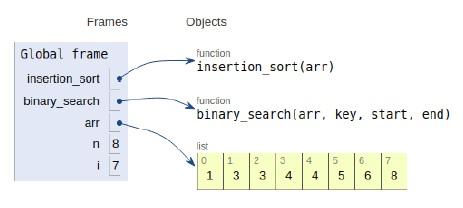# Python Program for Binary Insertion Sort

Problem statement − We are given an array, we need to sort it using the concept of binary insertion sort.

Here as the name suggests, we use the concept of the binary search along with the insertion sort algorithm.

Now let’s observe the solution in the implementation below −

## Example

Live Demo

# sort
def insertion_sort(arr):
for i in range(1, len(arr)):
temp = arr[i]
pos = binary_search(arr, temp, 0, i) + 1
for k in range(i, pos, -1):
arr[k] = arr[k - 1]
arr[pos] = temp
def binary_search(arr, key, start, end):
#key
if end - start <= 1:
if key < arr[start]:
return start - 1
else:
return start
mid = (start + end)//2
if arr[mid] < key:
return binary_search(arr, key, mid, end)
elif arr[mid] > key:
return binary_search(arr, key, start, mid)
else:
return mid
# main
arr = [1,5,3,4,8,6,3,4]
n = len(arr)
insertion_sort(arr)
print("Sorted array is:")
for i in range(n):
print(arr[i],end=" ")

## Output

Sorted array is :
1 3 3 4 4 5 5 6 8All the variables are declared in the local scope and their references are seen in the figure above.

## Conclusion

In this article, we have learned about how we can make a Python Program for Binary Insertion Sort